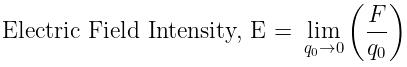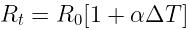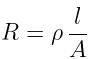Courses

Test: Electricity - 3

25 Questions MCQ Test General Science(Prelims) by IRS Divey Sethi | Test: Electricity - 3

Description
Attempt Test: Electricity - 3 | 25 questions in 25 minutes | Mock test for UPSC preparation | Free important questions MCQ to study General Science(Prelims) by IRS Divey Sethi for UPSC Exam | Download free PDF with solutions
QUESTION: 1

The space around a charge in which its effect can be felt is called its

Solution:

The electric field is the region around a charged particle where the lines of force can be felt by another charge by getting repulsed or attracted as per their sign of charges.

QUESTION: 2

The force acting on a unit positive test charge at a point inside an electric field is called

Solution:

Electric Field Intensity (E): The electrostatic force acting per unit positive charge on a point in an electric field is called electric field intensity at that point.QUESTION: 3

Work done in moving a unit positive test charge from infinity to a point inside an electric field, is called

Solution:

Electric potential: An electric potential is defined as the amount of electric potential energy that a unitary point electric charge would have if located at any point of space.
It is equal to the work done by an electric field in carrying a unit positive charge from infinity to that point.

QUESTION: 4

Work done is moving a unit positive test charge from one point to other inside an electric field, is called

Solution:

Potential difference is the difference in electric potential energy between two points.
It is the amount of work done in moving a unit positive charge from one point to another point in an electric field. This is measured in volt.

QUESTION: 5

Electricity constituted by electric charges at rest on the surface of a conductor, is called

Solution:

Static electricity is an imbalance of electric charges within or on the surface of a material. The charge remains until it is able to move away by means of an electric current or electrical discharge.

QUESTION: 6

Electricity constituted by moving electric charges, is called

Solution:

Electricity constituted by moving electric charge is called current.

QUESTION: 7

Time rate of flow of electric charge measures electric _________.

Solution:

Current is defined as the amount of electric charge flowing through a cross-section of circuits in unit time.
I = Q/T

QUESTION: 8

The condition for an electric charge to flow from one point to other is that the two points must have electric

Solution:

As we know the current is the flow of charges so it requires a potential difference between the two points.

QUESTION: 9

The device that maintains electric potential difference between two points is called electric

Solution:

Cell is the device which is used to maintain the potential difference between two points of the conductor.

QUESTION: 10

The path connecting two points at different potentials, to make the electric charges flow, is called electric _________.

Solution:

An electric circuit is the representation of the whole path of current and also the various devices connected in it, like Cell, Resistor, etc.

QUESTION: 11

The device which measures electric potential difference between two points is called

Solution:

A voltmeter is an instrument used for measuring the electrical potential difference between two points in an electric circuit.

QUESTION: 12

The device which measures electric current through a conductor is called

Solution:

Ammeter is used to measure electric current because it has very low resistance and are always connected in series

QUESTION: 13

Electric current is produced by flow of

Solution:

An electric current is said to exist when there is a net flow of electric charge through a region. In electric circuits this charge is often carried by electrons moving through a wire.

QUESTION: 14

Direction of flow of conventional current is taken from

Solution:

Conventional current or simply current, behaves as if positive charge carriers cause current to flow. Conventional current flows from the positive terminal to the negative.

QUESTION: 15

The law which gives a relation between electric potential difference and electric current is called

Solution:

Ohm's law states that the current through a conductor between two points is directly proportional to the voltage across the two points.

QUESTION: 16

With increase in temperature, resistance of a conductor

Solution:

As the temperature of a metallic conductor increases, the kinetic energy of the electrons of the conductor also increases
Due to this increase in kinetic energy more obstruction is offered to the flowing electrons i.e. current.
Therefore, as more obstruction is offered to the flowing electrons, the resistance is increased.QUESTION: 17

In series combination, total resistance

Solution:

When two or more resistors are connected in series, the total resistance of the combination is equal to the sum of all the individual resistances.

Thus, the total resistance of the circuit will always increase.

QUESTION: 18

In parallel combination, total resistance

Solution:

To find the total resistance of all components, the reciprocals of the resistances of each component are added and the reciprocal of the sum is taken.

Thus, the Total resistance will always be less than the value of the smallest resistance.

QUESTION: 19

In series combination, resistance increases due to increase in

Solution:where,
R = resistance,
ρ = resistivity,
ℓ = length,
A = area of cross-section

From the above relation: R ∝ ℓ
⇒ resistance increases due to an increase in length
Because the electrons have suffered greater collisions with atoms in the material.

QUESTION: 20

In parallel combination, resistance decreases due to increase in

Solution:

In parallel combination, change in length will not be effective, therefore we consider the area of cross-section.
As we know that resistance is inversely proportional to the area of cross-section.

Thus, resistance will decrease with an increase in area of crosssection.

QUESTION: 21

When electric current is passed, electrons move from

Solution:

Since electrons are negative they should be repelled by the negative charge and attracted by the positive charge, i.e., in an electric field the electrons must move from regions of low potential to the regions with high potential by the action of the electrical forces.

QUESTION: 22

Electrical resistivity of any given metallic wire depends upon

Solution:

Electrical resistivity of a given metallic wire depends on number density of free electrons in the conductor which is the nature of material

QUESTION: 23

In an atom, particle having a positive charge is called

Solution:

A positively charged subatomic particle that is housed within the nucleus is caled Proton.

QUESTION: 24

In an atom, particle having a negative charge, is called

Solution:

A negatively charged subatomic particle that has negligible mass and moves around in a cloud around the nucleus is called Electron.

QUESTION: 25

Substances whose atoms have more free electrons, are called

Solution:

Materials with high electron mobility (many free electrons) are called conductors, while materials with low electron mobility (few or no free electrons) are called insulators.Use Code STAYHOME200 and get INR 200 additional OFF Use Coupon Code

How to Prepare for UPSC

Read our guide to prepare for UPSC which is created by Toppers & the best Teachers

Track your progress, build streaks, highlight & save important lessons and more!

Similar ContentRelated tests